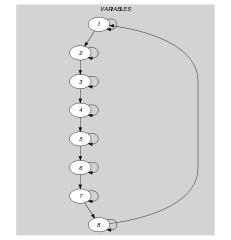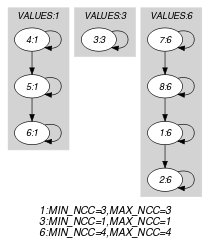## 5.375. stretch_circuit

Origin
Constraint

$\mathrm{𝚜𝚝𝚛𝚎𝚝𝚌𝚑}_\mathrm{𝚌𝚒𝚛𝚌𝚞𝚒𝚝}\left(\mathrm{𝚅𝙰𝚁𝙸𝙰𝙱𝙻𝙴𝚂},\mathrm{𝚅𝙰𝙻𝚄𝙴𝚂}\right)$

Usual name

$\mathrm{𝚜𝚝𝚛𝚎𝚝𝚌𝚑}$

Arguments
 $\mathrm{𝚅𝙰𝚁𝙸𝙰𝙱𝙻𝙴𝚂}$ $\mathrm{𝚌𝚘𝚕𝚕𝚎𝚌𝚝𝚒𝚘𝚗}\left(\mathrm{𝚟𝚊𝚛}-\mathrm{𝚍𝚟𝚊𝚛}\right)$ $\mathrm{𝚅𝙰𝙻𝚄𝙴𝚂}$ $\mathrm{𝚌𝚘𝚕𝚕𝚎𝚌𝚝𝚒𝚘𝚗}\left(\mathrm{𝚟𝚊𝚕}-\mathrm{𝚒𝚗𝚝},\mathrm{𝚕𝚖𝚒𝚗}-\mathrm{𝚒𝚗𝚝},\mathrm{𝚕𝚖𝚊𝚡}-\mathrm{𝚒𝚗𝚝}\right)$
Restrictions
 $|\mathrm{𝚅𝙰𝚁𝙸𝙰𝙱𝙻𝙴𝚂}|>0$ $\mathrm{𝚛𝚎𝚚𝚞𝚒𝚛𝚎𝚍}$$\left(\mathrm{𝚅𝙰𝚁𝙸𝙰𝙱𝙻𝙴𝚂},\mathrm{𝚟𝚊𝚛}\right)$ $|\mathrm{𝚅𝙰𝙻𝚄𝙴𝚂}|>0$ $\mathrm{𝚛𝚎𝚚𝚞𝚒𝚛𝚎𝚍}$$\left(\mathrm{𝚅𝙰𝙻𝚄𝙴𝚂},\left[\mathrm{𝚟𝚊𝚕},\mathrm{𝚕𝚖𝚒𝚗},\mathrm{𝚕𝚖𝚊𝚡}\right]\right)$ $\mathrm{𝚍𝚒𝚜𝚝𝚒𝚗𝚌𝚝}$$\left(\mathrm{𝚅𝙰𝙻𝚄𝙴𝚂},\mathrm{𝚟𝚊𝚕}\right)$ $\mathrm{𝚅𝙰𝙻𝚄𝙴𝚂}.\mathrm{𝚕𝚖𝚒𝚗}\le \mathrm{𝚅𝙰𝙻𝚄𝙴𝚂}.\mathrm{𝚕𝚖𝚊𝚡}$ $\mathrm{𝚅𝙰𝙻𝚄𝙴𝚂}.\mathrm{𝚕𝚖𝚒𝚗}\le |\mathrm{𝚅𝙰𝚁𝙸𝙰𝙱𝙻𝙴𝚂}|$ $\mathrm{𝚜𝚞𝚖}$$\left(\mathrm{𝚅𝙰𝙻𝚄𝙴𝚂}.\mathrm{𝚕𝚖𝚒𝚗}\right)\le |\mathrm{𝚅𝙰𝚁𝙸𝙰𝙱𝙻𝙴𝚂}|$
Purpose

In order to define the meaning of the $\mathrm{𝚜𝚝𝚛𝚎𝚝𝚌𝚑}_\mathrm{𝚙𝚊𝚝𝚑}$ constraint, we first introduce the notions of stretch and span. Let $n$ be the number of variables of the collection $\mathrm{𝚅𝙰𝚁𝙸𝙰𝙱𝙻𝙴𝚂}$ and let $i$, $j$ $\left(0\le i be two positions within the collection of variables $\mathrm{𝚅𝙰𝚁𝙸𝙰𝙱𝙻𝙴𝚂}$ such that the following conditions apply:

• If $i\le j$ then all variables ${X}_{i},\cdots ,{X}_{j}$ take a same value from the set of values of the $\mathrm{𝚟𝚊𝚕}$ attribute.

If $i>j$ then all variables ${X}_{i},\cdots ,{X}_{n-1},{X}_{0},\cdots ,{X}_{j}$ take a same value from the set of values of the $\mathrm{𝚟𝚊𝚕}$ attribute.

• ${X}_{\left(i-1\right)modn}$ is different from ${X}_{i}$.

• ${X}_{\left(j+1\right)modn}$ is different from ${X}_{j}$.

We call such a set of variables a stretch. The span of the stretch is equal to $1+\left(j-i\right)modn$, while the value of the stretch is ${X}_{i}$. We now define the condition enforced by the $\mathrm{𝚜𝚝𝚛𝚎𝚝𝚌𝚑}_\mathrm{𝚌𝚒𝚛𝚌𝚞𝚒𝚝}$ constraint.

Each item $\left(\mathrm{𝚟𝚊𝚕}-v,\mathrm{𝚕𝚖𝚒𝚗}-s,\mathrm{𝚕𝚖𝚊𝚡}-t\right)$ of the $\mathrm{𝚅𝙰𝙻𝚄𝙴𝚂}$ collection enforces the minimum value $s$ as well as the maximum value $t$ for the span of a stretch of value $v$.

Note that:

1. Having an item $\left(\mathrm{𝚟𝚊𝚕}-v,\mathrm{𝚕𝚖𝚒𝚗}-s,\mathrm{𝚕𝚖𝚊𝚡}-t\right)$ with $s$ strictly greater than 0 does not mean that value $v$ should be assigned to one of the variables of collection $\mathrm{𝚅𝙰𝚁𝙸𝙰𝙱𝙻𝙴𝚂}$. It rather means that, when value $v$ is used, all stretches of value $v$ must have a span that belong to interval $\left[s,t\right]$.

2. A variable of the collection $\mathrm{𝚅𝙰𝚁𝙸𝙰𝙱𝙻𝙴𝚂}$ may be assigned a value that is not defined in the $\mathrm{𝚅𝙰𝙻𝚄𝙴𝚂}$ collection.

Example
$\left(\begin{array}{c}〈6,6,3,1,1,1,6,6〉,\hfill \\ 〈\begin{array}{ccc}\mathrm{𝚟𝚊𝚕}-1\hfill & \mathrm{𝚕𝚖𝚒𝚗}-2\hfill & \mathrm{𝚕𝚖𝚊𝚡}-4,\hfill \\ \mathrm{𝚟𝚊𝚕}-2\hfill & \mathrm{𝚕𝚖𝚒𝚗}-2\hfill & \mathrm{𝚕𝚖𝚊𝚡}-3,\hfill \\ \mathrm{𝚟𝚊𝚕}-3\hfill & \mathrm{𝚕𝚖𝚒𝚗}-1\hfill & \mathrm{𝚕𝚖𝚊𝚡}-6,\hfill \\ \mathrm{𝚟𝚊𝚕}-6\hfill & \mathrm{𝚕𝚖𝚒𝚗}-2\hfill & \mathrm{𝚕𝚖𝚊𝚡}-4\hfill \end{array}〉\hfill \end{array}\right)$

The $\mathrm{𝚜𝚝𝚛𝚎𝚝𝚌𝚑}_\mathrm{𝚌𝚒𝚛𝚌𝚞𝚒𝚝}$ constraint holds since the sequence $66311166$ contains three stretches $6666$, 3, and $111$ respectively verifying the following conditions:

• The span of the first stretch $6666$ is located within interval $\left[2,4\right]$ (i.e., the limit associated with value 6).

• The span of the second stretch 3 is located within interval $\left[1,6\right]$ (i.e., the limit associated with value 3).

• The span of the third stretch $111$ is located within interval $\left[2,4\right]$ (i.e., the limit associated with value 1).

Typical
 $|\mathrm{𝚅𝙰𝚁𝙸𝙰𝙱𝙻𝙴𝚂}|>1$ $\mathrm{𝚛𝚊𝚗𝚐𝚎}$$\left(\mathrm{𝚅𝙰𝚁𝙸𝙰𝙱𝙻𝙴𝚂}.\mathrm{𝚟𝚊𝚛}\right)>1$ $|\mathrm{𝚅𝙰𝚁𝙸𝙰𝙱𝙻𝙴𝚂}|>|\mathrm{𝚅𝙰𝙻𝚄𝙴𝚂}|$ $|\mathrm{𝚅𝙰𝙻𝚄𝙴𝚂}|>1$ $\mathrm{𝚅𝙰𝙻𝚄𝙴𝚂}.\mathrm{𝚕𝚖𝚊𝚡}\le |\mathrm{𝚅𝙰𝚁𝙸𝙰𝙱𝙻𝙴𝚂}|$
Symmetries
• Items of $\mathrm{𝚅𝙰𝚁𝙸𝙰𝙱𝙻𝙴𝚂}$ can be shifted.

• Items of $\mathrm{𝚅𝙰𝙻𝚄𝙴𝚂}$ are permutable.

• All occurrences of two distinct values in $\mathrm{𝚅𝙰𝚁𝙸𝙰𝙱𝙻𝙴𝚂}.\mathrm{𝚟𝚊𝚛}$ or $\mathrm{𝚅𝙰𝙻𝚄𝙴𝚂}.\mathrm{𝚟𝚊𝚕}$ can be swapped; all occurrences of a value in $\mathrm{𝚅𝙰𝚁𝙸𝙰𝙱𝙻𝙴𝚂}.\mathrm{𝚟𝚊𝚛}$ or $\mathrm{𝚅𝙰𝙻𝚄𝙴𝚂}.\mathrm{𝚟𝚊𝚕}$ can be renamed to any unused value.

Usage

The article [Pesant01], which originally introduced the $\mathrm{𝚜𝚝𝚛𝚎𝚝𝚌𝚑}$ constraint, quotes rostering problems as typical examples of use of this constraint.

Remark

We split the origin $\mathrm{𝚜𝚝𝚛𝚎𝚝𝚌𝚑}$ constraint into the $\mathrm{𝚜𝚝𝚛𝚎𝚝𝚌𝚑}_\mathrm{𝚌𝚒𝚛𝚌𝚞𝚒𝚝}$ and the $\mathrm{𝚜𝚝𝚛𝚎𝚝𝚌𝚑}_\mathrm{𝚙𝚊𝚝𝚑}$ constraints that respectively use the $\mathrm{𝑃𝐴𝑇𝐻}$ $\mathrm{𝐿𝑂𝑂𝑃}$ and $\mathrm{𝐶𝐼𝑅𝐶𝑈𝐼𝑇}$ $\mathrm{𝐿𝑂𝑂𝑃}$ arc generators. We also reorganise the parameters: the $\mathrm{𝚅𝙰𝙻𝚄𝙴𝚂}$ collection describes the attributes of each value that can be assigned to the variables of the $\mathrm{𝚜𝚝𝚛𝚎𝚝𝚌𝚑}_\mathrm{𝚌𝚒𝚛𝚌𝚞𝚒𝚝}$ constraint. Finally we skipped the pattern constraint that tells what values can follow a given value.

Algorithm

A first filtering algorithm was described in the original article of G. Pesant [Pesant01]. An algorithm that also generates explanations is given in [RochartJussien03]. The first filtering algorithm achieving arc-consistency is depicted in [Hellsten04], [HellstenPesantBeek04]. This algorithm is based on dynamic programming and handles the fact that some values can be followed by only a given subset of values.

Reformulation

The $\mathrm{𝚜𝚝𝚛𝚎𝚝𝚌𝚑}_\mathrm{𝚌𝚒𝚛𝚌𝚞𝚒𝚝}$ constraint can be reformulated in term of a $\mathrm{𝚜𝚝𝚛𝚎𝚝𝚌𝚑}_\mathrm{𝚙𝚊𝚝𝚑}$ constraint. Let $\mathrm{𝐿𝑀𝐴𝑋}$ denote the maximum value taken by the $\mathrm{𝚕𝚖𝚊𝚡}$ attribute within the items of the collection $\mathrm{𝚅𝙰𝙻𝚄𝙴𝚂}$, let $n$ be the number of variables of the collection $\mathrm{𝚅𝙰𝚁𝙸𝙰𝙱𝙻𝙴𝚂}$, and let $\delta =min\left(\mathrm{𝐿𝑀𝐴𝑋},n\right)$. The first and second arguments of the $\mathrm{𝚜𝚝𝚛𝚎𝚝𝚌𝚑}_\mathrm{𝚙𝚊𝚝𝚑}$ constraint are created in the following way:

Even if $\mathrm{𝚜𝚝𝚛𝚎𝚝𝚌𝚑}_\mathrm{𝚙𝚊𝚝𝚑}$ can achieve arc-consistency this reformulation may not achieve arc-consistency since it duplicates variables.

Using this reformulation, the example

$\mathrm{𝚜𝚝𝚛𝚎𝚝𝚌𝚑}_\mathrm{𝚌𝚒𝚛𝚌𝚞𝚒𝚝}$$\left(〈6,6,3,1,1,1,6,6〉,$

$〈\mathrm{𝚟𝚊𝚕}-1\mathrm{𝚕𝚖𝚒𝚗}-2\mathrm{𝚕𝚖𝚊𝚡}-4,\mathrm{𝚟𝚊𝚕}-2\mathrm{𝚕𝚖𝚒𝚗}-2\mathrm{𝚕𝚖𝚊𝚡}-3,$

$\mathrm{𝚟𝚊𝚕}-3\mathrm{𝚕𝚖𝚒𝚗}-1\mathrm{𝚕𝚖𝚊𝚡}-6,\mathrm{𝚟𝚊𝚕}-6\mathrm{𝚕𝚖𝚒𝚗}-2\mathrm{𝚕𝚖𝚊𝚡}-4〉\right)$

of the Example slot is reformulated as:

$\mathrm{𝚜𝚝𝚛𝚎𝚝𝚌𝚑}_\mathrm{𝚙𝚊𝚝𝚑}$$\left(〈6,6,3,1,1,1,6,6,6,6,3,1,1,1〉,$

$〈\mathrm{𝚟𝚊𝚕}-1\mathrm{𝚕𝚖𝚒𝚗}-2\mathrm{𝚕𝚖𝚊𝚡}-4,\mathrm{𝚟𝚊𝚕}-2\mathrm{𝚕𝚖𝚒𝚗}-2\mathrm{𝚕𝚖𝚊𝚡}-3,$

$\mathrm{𝚟𝚊𝚕}-3\mathrm{𝚕𝚖𝚒𝚗}-1\mathrm{𝚕𝚖𝚊𝚡}-6,\mathrm{𝚟𝚊𝚕}-6\mathrm{𝚕𝚖𝚒𝚗}-2\mathrm{𝚕𝚖𝚊𝚡}-4〉\right)$

In the reformulation $\delta$ was equal to 6, and the $\mathrm{𝚅𝙰𝙻𝚄𝙴𝚂}$ collection was left unchanged since no $\mathrm{𝚕𝚖𝚊𝚡}$ attribute was equal to the number of variables of the $\mathrm{𝚅𝙰𝚁𝙸𝙰𝙱𝙻𝙴𝚂}$ collection (i.e., 8).

Keywords

For all items of $\mathrm{𝚅𝙰𝙻𝚄𝙴𝚂}$:

Arc input(s)

$\mathrm{𝚅𝙰𝚁𝙸𝙰𝙱𝙻𝙴𝚂}$

Arc generator
 $\mathrm{𝐶𝐼𝑅𝐶𝑈𝐼𝑇}$$↦\mathrm{𝚌𝚘𝚕𝚕𝚎𝚌𝚝𝚒𝚘𝚗}\left(\mathrm{𝚟𝚊𝚛𝚒𝚊𝚋𝚕𝚎𝚜}\mathtt{1},\mathrm{𝚟𝚊𝚛𝚒𝚊𝚋𝚕𝚎𝚜}\mathtt{2}\right)$ $\mathrm{𝐿𝑂𝑂𝑃}$$↦\mathrm{𝚌𝚘𝚕𝚕𝚎𝚌𝚝𝚒𝚘𝚗}\left(\mathrm{𝚟𝚊𝚛𝚒𝚊𝚋𝚕𝚎𝚜}\mathtt{1},\mathrm{𝚟𝚊𝚛𝚒𝚊𝚋𝚕𝚎𝚜}\mathtt{2}\right)$

Arc arity
Arc constraint(s)
 $•\mathrm{𝚟𝚊𝚛𝚒𝚊𝚋𝚕𝚎𝚜}\mathtt{1}.\mathrm{𝚟𝚊𝚛}=\mathrm{𝚅𝙰𝙻𝚄𝙴𝚂}.\mathrm{𝚟𝚊𝚕}$ $•\mathrm{𝚟𝚊𝚛𝚒𝚊𝚋𝚕𝚎𝚜}\mathtt{2}.\mathrm{𝚟𝚊𝚛}=\mathrm{𝚅𝙰𝙻𝚄𝙴𝚂}.\mathrm{𝚟𝚊𝚕}$
Graph property(ies)
 $•$$\mathrm{𝚗𝚘𝚝}_\mathrm{𝚒𝚗}$$\left($$\mathrm{𝐌𝐈𝐍}_\mathrm{𝐍𝐂𝐂}$$,1,\mathrm{𝚅𝙰𝙻𝚄𝙴𝚂}.\mathrm{𝚕𝚖𝚒𝚗}-1\right)$ $•$$\mathrm{𝐌𝐀𝐗}_\mathrm{𝐍𝐂𝐂}$$\le \mathrm{𝚅𝙰𝙻𝚄𝙴𝚂}.\mathrm{𝚕𝚖𝚊𝚡}$

Graph model

Part (A) of Figure 5.375.1 shows the initial graphs associated with values 1, 2, 3 and 6 of the Example slot. Part (B) of Figure 5.375.1 shows the corresponding final graphs associated with values 1, 3 and 6. Since value 2 is not assigned to any variable of the $\mathrm{𝚅𝙰𝚁𝙸𝙰𝙱𝙻𝙴𝚂}$ collection the final graph associated with value 2 is empty. The $\mathrm{𝚜𝚝𝚛𝚎𝚝𝚌𝚑}_\mathrm{𝚌𝚒𝚛𝚌𝚞𝚒𝚝}$ constraint holds since:

• For value 1 we have one connected component for which the number of vertices is greater than or equal to 2 and less than or equal to 4,

• For value 2 we do not have any connected component,

• For value 3 we have one connected component for which the number of vertices is greater than or equal to 1 and less than or equal to 6,

• For value 6 we have one connected component for which the number of vertices is greater than or equal to 2 and less than or equal to 4.

##### Figure 5.375.1. Initial and final graph of the $\mathrm{𝚜𝚝𝚛𝚎𝚝𝚌𝚑}_\mathrm{𝚌𝚒𝚛𝚌𝚞𝚒𝚝}$ constraint(a) (b)